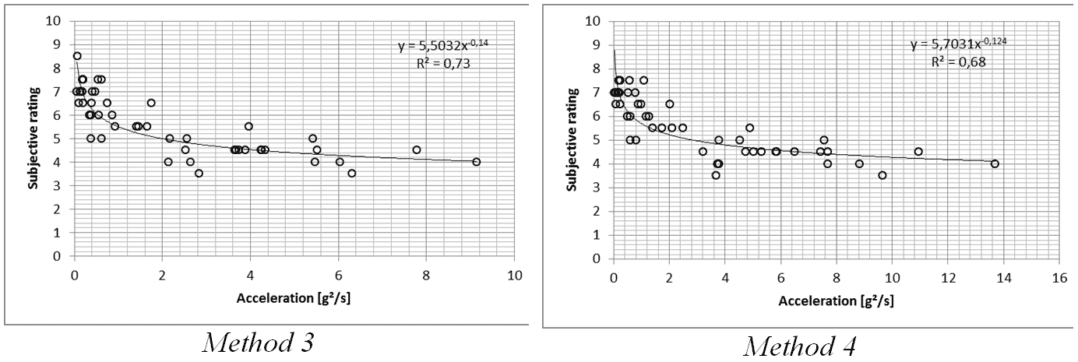# TBR Technical Corner: Study of Correlation between Subjective and Objective Results in a Brake Judder DTV Sensitivity Test (Part 2 of 3)

Part 2 of 3. Read Part 1 here.

In the first part of the article, the study aimed at correlating subjective ratings and objective acceleration results gathered under operational judder was presented.

Vibrations are first pre-processed using a series of human perception filters; then, the impulsive root mean square (IRMS) of the vibration perceived by the driver is calculated for different window sizes. The judder phenomenon is finally quantified using different methods, as explained in the following paragraphs.

#### Objective Analysis Methods

In order to compare the subjective rating with the objective measurements, a single value of acceleration must be calculated. The calculation methods used in this study are:

Method 1: Maximum peak acceleration

The first correlation study is performed using the maximum value of the IRMS for each snub. This method does not take into account the duration of the judder, but the highest level it reaches. It is assumed that drivers usually value more the intensity of the vibration rather than its duration.

Method 2: Integrated squared acceleration by unit time

The second method used integrates the square of the IRMS curve. The square of the acceleration enlarges the judder peaks, while integrating the curve allows the duration of the judder peak to be taken into account. The integrated amplitude is then divided by the time of the snub for normalization. Note that amplitudes are in g2.Method 3: Integrate above 50% of maximum peak

The third method integrates the peaks. Like this, if the judder is longer, the integrated amplitude should be higher. Two methods have been studied (methods 3 and 4 in this study); the first one is to integrate the amplitude above 50% of the maximum peak of the snub. Snubs with higher peaks will have higher thresholds. For this method the IRMS vector is also squared to amplify peaks.

Method 4: Integrate above the minimum maximum peak

In order to have the same threshold level for the integration of all the snubs, this threshold is the lowest maximum peak of all the snubs performed. For that snub the integration gives 0 as there is nothing higher than its maximum. For this method the IRMS vector is also squared to amplify peaks.

#### Results

The correlation between subjective rating and objective results are calculated using the coefficient of determination R squared. For the first method a regression line is used. For the other methods, a power regression is used because the signal has been squared.

Steering wheel analysis

The correlation results for the steering wheel are summarized below:

 Window(s) Method 1 Method 2 Method 3 Method 4 0.35 0.73 0.73 0.75 0.56 0.5 0.73 0.74 0.74 0.56 0.75 0.74 0.73 0.73 0.68 1 0.78 0.72 0.73 0.68 1.25 0.75 0.73 0.73 0.68

The correlation is generally very satisfactory for all the methods and window lengths. The fourth method is the one with the lowest correlation. In general, the window length of 1 s shows the best correlation levels. As an example, the following figures show the results for each method presented for a single window length (1 s):Brake pedal analysis

The correlation results for the brake pedal are summarized below:

 Window(s) Method 1 Method 2 Method 3 Method 4 0.35 0.53 0.59 0.49 0.51 0.5 0.51 0.59 0.50 0.41 0.75 0.51 0.59 0.50 0.45 1 0.51 0.59 0.51 0.60 1.25 0.51 0.58 0.51 0.62

The correlation for the brake pedal is generally poor. Only method 2 and method 4 have R squared ≈ 0.6. Brake pedal is more difficult to correlate due to the fact that the foot is pressing the pedal focused on modulating the deceleration. In general, the higher correlations are found with the second method (integrate square of IRMS by unit time). The 0.75s window gives better overall results.Having introduced the scope of the study, explained the methodology and (partially) seen the results, the next chapter will present the main conclusions from this work.